Comparing 3-Digit Numbers Math Video for Kids | K, 1st, & 2nd Grade
1%
It was processed successfully!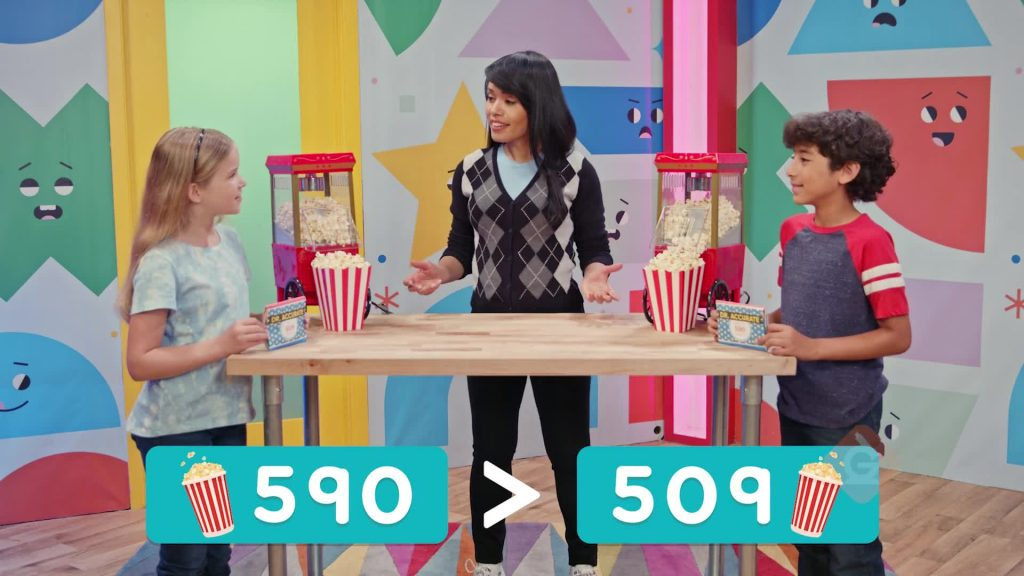WHAT IS COMPARING 3-DIGIT NUMBERS?

Students learn to compare two 3-digit numbers. They express comparisons using the equal sign and the inequality signs > and <.

To better understand comparing 3-digit numbers…

WHAT IS COMPARING 3-DIGIT NUMBERS?. Students learn to compare two 3-digit numbers. They express comparisons using the equal sign and the inequality signs > and <. To better understand comparing 3-digit numbers…

## LET’S BREAK IT DOWN!

### Review Place Value – Cheese Puffs!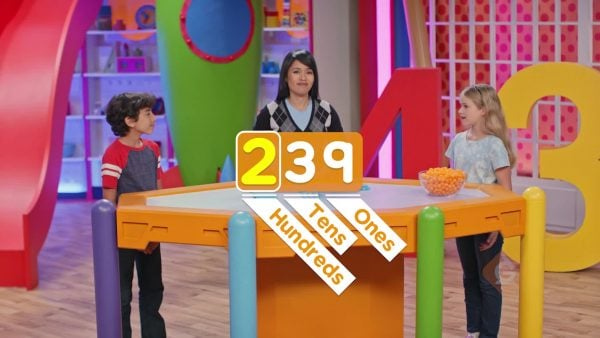Place value is the value of a digit based on its position in a number. A bowl has 239 cheese puffs. Break apart 239 using place value. 239 = 2 hundreds + 3 tens + 9 ones. You can represent this number using base-10 blocks. What if you add 1 more ones block to 239? Now you have 10 ones. 10 ones is the same as 1 ten. So now you have 2 hundreds, 4 tens, and 0 ones. This is the number 240. Try this one yourself: How can you show the number 354 using base-10 blocks?

Review Place Value – Cheese Puffs! Place value is the value of a digit based on its position in a number. A bowl has 239 cheese puffs. Break apart 239 using place value. 239 = 2 hundreds + 3 tens + 9 ones. You can represent this number using base-10 blocks. What if you add 1 more ones block to 239? Now you have 10 ones. 10 ones is the same as 1 ten. So now you have 2 hundreds, 4 tens, and 0 ones. This is the number 240. Try this one yourself: How can you show the number 354 using base-10 blocks?

### Hundreds Place – Dance Video Game!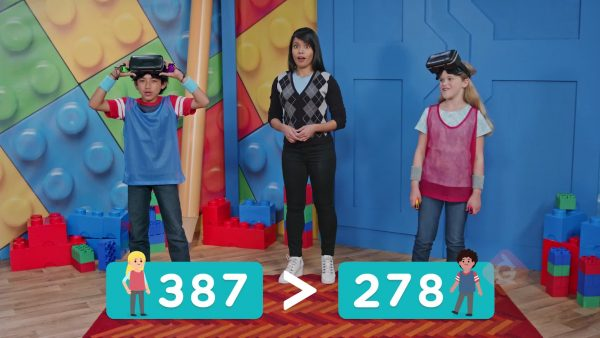Madeleine has 387 points. Omr has 278 points. Who is winning? Compare 387 and 278 using place value. Start by comparing the digits in the greatest place value, the hundreds. 3 hundreds is greater than 2 hundreds. So, 387 is greater than 278, which means Madeleine is winning. You can write this using the greater than symbol: 387 > 278. The symbol is like an alligator’s mouth. It always wants to eat the greater number. Now Madeleine has 438 points. Omr has 456 points. Who is winning now? 438 and 456 both have a 4 in the hundreds place. Compare the tens place. 3 tens is less than 5 tens. So, 438 is less than 456, and Omr is winning. You can write this using the less than symbol: 438 < 456. At the end of the game, Omr has 529 points. Madeleine has 527 points. 529 and 527 both have a 5 in the hundreds place. 529 and 527 both have a 2 in the tens place. Compare the ones place. 9 is greater than 7. So, 529 is greater than 527, and Omr wins the game. Try this one yourself: After a bonus round, Madeleine has 648 points. Omr has 619 points. Who has more points?

Hundreds Place – Dance Video Game! Madeleine has 387 points. Omr has 278 points. Who is winning? Compare 387 and 278 using place value. Start by comparing the digits in the greatest place value, the hundreds. 3 hundreds is greater than 2 hundreds. So, 387 is greater than 278, which means Madeleine is winning. You can write this using the greater than symbol: 387 > 278. The symbol is like an alligator’s mouth. It always wants to eat the greater number. Now Madeleine has 438 points. Omr has 456 points. Who is winning now? 438 and 456 both have a 4 in the hundreds place. Compare the tens place. 3 tens is less than 5 tens. So, 438 is less than 456, and Omr is winning. You can write this using the less than symbol: 438 < 456. At the end of the game, Omr has 529 points. Madeleine has 527 points. 529 and 527 both have a 5 in the hundreds place. 529 and 527 both have a 2 in the tens place. Compare the ones place. 9 is greater than 7. So, 529 is greater than 527, and Omr wins the game. Try this one yourself: After a bonus round, Madeleine has 648 points. Omr has 619 points. Who has more points?

### Tens Place – Balloon Cars!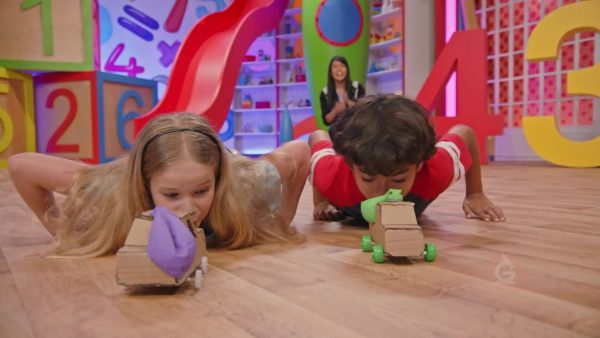Madeleine and Omr are racing balloon cars. Madeleine’s car went 184 cm. Omr’s car went 91 cm. Whose car traveled farther? Compare the two numbers using place value. Start in the hundreds place. Remember that you always need to compare the same place value in both numbers. In 91, the 9 is in the tens place. You can put a zero in the hundreds place next to 91. The zero does not change the value of the number. 184 has a 1 in the hundreds place and 91 has a 0 in the hundreds place. 1 is greater than 0. So, 184 is greater than 91. Madeleine’s car traveled farther. Try this one yourself: On the next race, Madeleine’s car went 87 cm. Omr’s car went 154 cm. Whose car traveled farther?

Tens Place – Balloon Cars! Madeleine and Omr are racing balloon cars. Madeleine’s car went 184 cm. Omr’s car went 91 cm. Whose car traveled farther? Compare the two numbers using place value. Start in the hundreds place. Remember that you always need to compare the same place value in both numbers. In 91, the 9 is in the tens place. You can put a zero in the hundreds place next to 91. The zero does not change the value of the number. 184 has a 1 in the hundreds place and 91 has a 0 in the hundreds place. 1 is greater than 0. So, 184 is greater than 91. Madeleine’s car traveled farther. Try this one yourself: On the next race, Madeleine’s car went 87 cm. Omr’s car went 154 cm. Whose car traveled farther?

### Ones Place – Popcorn!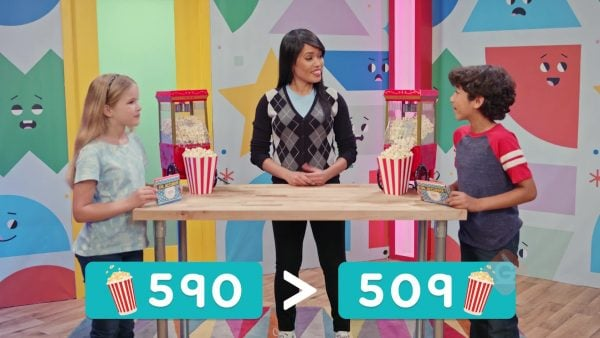Omr has a bag of 590 popcorn kernels. Madeleine has a bag of 509 popcorn kernels. Who has more popcorn kernels? Compare the amounts by place value. Start with the greatest place value, the hundreds place. Both numbers have a 5 in the hundreds place. Compare the digits in the tens place. 590 has a 9 in the tens place. 509 has a 0 in the tens place. 9 is greater than 0. So, 590 is greater than 509. Omr has more popcorn kernels. Try this one yourself: Omr has a bag of 638 raisins. Madeleine has a bag of 683 raisins. Who has more raisins?

Ones Place – Popcorn! Omr has a bag of 590 popcorn kernels. Madeleine has a bag of 509 popcorn kernels. Who has more popcorn kernels? Compare the amounts by place value. Start with the greatest place value, the hundreds place. Both numbers have a 5 in the hundreds place. Compare the digits in the tens place. 590 has a 9 in the tens place. 509 has a 0 in the tens place. 9 is greater than 0. So, 590 is greater than 509. Omr has more popcorn kernels. Try this one yourself: Omr has a bag of 638 raisins. Madeleine has a bag of 683 raisins. Who has more raisins?

## COMPARING 3-DIGIT NUMBERS VOCABULARY

Place value
The value of a digit based on its position in a number.
Compare
When you compare two numbers, you find which number is greater and which number is lesser.
>
If the value of one number is more than the other, we say it is greater than. This symbol shows that the number on the left is greater. 40 > 10.
<
If the value of one number is smaller than the other, it is less than. This symbol shows the number on the left is less. 10 < 40.
=
When comparing two numbers, if the values are the same, we say they are equal. This symbol means the quantities on both sides are equal. 40 = 40.
Expanded Form
Shows the number as a sum of the value of the digits. For example, 234 = 200 + 30 + 4.

## COMPARING 3-DIGIT NUMBERS DISCUSSION QUESTIONS

### What process do you use to compare numbers?

I first compare the digits in the greatest place value. If the digits are the same, I compare the next greatest place value. For example, to compare 604 and 356, I first compare 6 and 3. 6 > 3, so 604 > 356.

### How can you compare 156 and 187?

The digits in the hundreds place are the same. Next, compare the digits in the tens place. 5 is less than 8, so 156 is less than 187.

### How can you compare 672 and 670?

The digits in the hundreds and tens places are the same. Next, compare the digits in the ones place. 2 is greater than 0, so 672 is greater than 670.

### How can you compare 234 and 97?

Compare the digits in the hundreds place. 234 has a 2 in the hundreds place. 97 has no hundreds, so it has a 0 in the hundreds place. 2 is greater than 0, so 234 is greater than 97.

### When you use the greater than and less than symbols to compare numbers, how do you know which one to use?

The open side of the symbol always faces the greater number. It is like an alligator mouth that always wants to eat the greater number.
X

## Success

We’ve sent you an email with instructions how to reset your password.
Ok